# Enthalpy

Statistical mechanics$S = k_B \, \ln\Omega$
Statistical thermodynamics
Kinetic theory
Potentials
Internal energy ·Enthalpy
Helmholtz free energy
Gibbs free energy

In thermodynamics and molecular chemistry, the enthalpy or heat content (denoted as H, h, or rarely as χ) is a quotient or description of thermodynamic potential of a system, which can be used to calculate the "useful" work obtainable from a closed thermodynamic system under constant pressure and entropy.

## Etymology

The term enthalpy was composed of the prefix en-, meaning to "put into" and the Greek word -thalpein, meaning "to heat," although the original definition is thought to have stemmed from the word "enthalpos" (ἐνθάλπος).

## History

Over the history of thermodynamics, several terms have been used to denote what is now known as the enthalpy of a system. Originally, it was thought that the word "enthalpy" was created by Benoit Paul Émile Clapeyron and Rudolf Clausius through the publishing of the Clausius-Clapeyron relation in The Mollier Steam Tables and Diagrams in 1827, but it was later published that the earliest recording of the word was in 1875, by Josiah Willard Gibbs in the publication Physical Chemistry: an Advanced Treatise, although it is not referenced in Gibbs' works directly. In 1909, Keith Landler discussed Gibbs' work on the 'heat function for constant pressure' and noted that Heike Kamerlingh Onnes had coined its modern name from the Greek word "enthalpos" (ενθαλπος) meaning "to put heat into."

### Original definition

This is the heat change which occurs when 1 mol of a substance reacts completely with oxygen to form products at 298 K and 1 atm. The function H was introduced by the Dutch physicist Kamerlingh Onnes in the early twentieth century in the following form: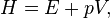$H = E + pV,\,$

where E represents the energy of the system. In the absence of an external field, the enthalpy may be defined, as it is generally known, by: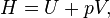$H = U + pV,\,$

where (all units given in SI)

• H is the enthalpy (joules)
• U is the internal energy, (joules)
• p is the pressure of the system, (pascals)
• V is the volume, (cubic metres)

## Application and extended formula

### Overview

In terms of thermodynamics, enthalpy can be calculated by determining the requirements for creating a system from "nothingness"; the mechanical work required,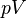$pV$ differs, based upon the constancy of conditions present at the creation of the thermodynamic system.

Internal energy,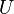$U$, must be supplied to remove particles from a surrounding in order to allow space for the creation of a system, providing that environmental variables, such as pressure ($p$) remain constant. This internal energy also includes the energy required for activation and the breaking of bonded compounds into gaseous species.

This process is calculated within enthalpy calculations as$U + pV$, to label the amount of energy or work required to "set aside space for" and "create" the system; describing the work done by both the reaction or formation of systems, and the surroundings. For systems at constant pressure, the change in enthalpy is the heat received by the system plus the non-mechanical work that has been done.

Therefore, the change in enthalpy can be devised or represented without the need for compressive or expansive mechanics; for a simple system, with a constant number of particles, the difference in enthalpy is the maximum amount of thermal energy derivable from a thermodynamic process in which the pressure is held constant.

The term$p$$V$ is the work required to displace the surrounding atmosphere in order to vacate the space to be occupied by the system.

### Relationships

As an expansion of the first law of thermodynamics, enthalpy can be related to several other thermodynamic formulae. As with the original definition of the first law;$\mathrm{d}U = \delta Q - \delta W\,$
Where, as defined by the law;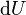$\mathrm{d}U$ represents the infinitesimal increase of the systematic or internal energy.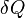$\delta Q$ represents the infinitesimal amount of energy attributed or added to the system.$\delta W$ represents the infinitesimal amount of energy acted out by the system on the surroundings.

As a differential expression, the value of H can be defined as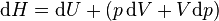$\mathrm{d}H = \mathrm{d}U + (p\,\mathrm{d}V + V\mathrm{d}p) \!$$= (\delta Q - p\,\mathrm{d}V) + (p\,\mathrm{d}V + V\mathrm{d}p) \!$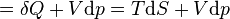$= \delta Q +V\mathrm{d}p = T\mathrm{d}S + V\mathrm{d}p \!$

Where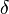$\delta$ represents the inexact differential$U$ is the internal energy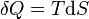$\delta Q=T\mathrm{d}S$ is the energy added by heating during a reversible process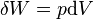$\delta W=p\mathrm{d}V$ is the work done by the system in a reversible process$\mathrm{d}S$ is the increase in entropy (joules per kelvin)$p$ is the constant pressure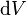$\mathrm{d}V$ is an infinitesimal volume$T$ is the temperature (kelvins)

For a process that is not reversible, the above equation expressing dH in terms of dS and dP still holds because H is a thermodynamic state variable that can be uniquely specified by S and P. We thus have in general: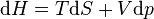$\mathrm{d}H = T\mathrm{d}S+V\mathrm{d}p\,$

It is seen that, if a thermodynamic process is isobaric (i.e., occurs at constant pressure), then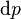$\mathrm{d}p$ is zero and thus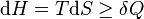$\mathrm{d}H = T\mathrm{d}S\geq \delta Q \,$

The difference in enthalpy is the maximum thermal energy attainable from the system in an isobaric process. This explains why it is sometimes called the heat content. That is, the integral of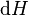$\mathrm{d}H$ over any isobar in state space is the maximum thermal energy attainable from the system.

In a more general form, the first law describes the internal energy with additional terms involving the chemical potential and the number of particles of various types. The differential statement for$\mathrm{d}H$  is then: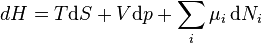$dH = T\mathrm{d}S+V\mathrm{d}p + \sum_i \mu_i \,\mathrm{d}N_i\,$

where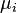$\mu_i$ is the chemical potential for an i-type particle, and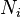$N_i$ is the number of such particles. It is seen that, not only must the$V\mathrm{d}p$  term be set to zero by requiring the pressures of the initial and final states to be the same, but the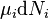$\mu_i \mathrm{d}N_i$ terms must be zero as well, by requiring that the particle numbers remain unchanged. Any further generalization will add even more terms whose extensive differential term must be set to zero in order for the interpretation of the enthalpy to hold.

### Heats of reaction

The total enthalpy of a system cannot be measured directly; the enthalpy change of a system is measured instead. Enthalpy change is defined by the following equation: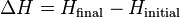$\Delta H = H_\mathrm{final} - H_\mathrm{initial} \,$

where

ΔH  is the enthalpy change
Hfinal is the final enthalpy of the system, measured in joules. In a chemical reaction, Hfinal is the enthalpy of the products.
Hinitial is the initial enthalpy of the system, measured in joules. In a chemical reaction, Hinitial is the enthalpy of the reactants.

For an exothermic reaction at constant pressure, the system's change in enthalpy is equal to the energy released in the reaction, including the energy retained in the system and lost through expansion against its surroundings. In a similar manner, for an endothermic reaction, the system's change in enthalpy is equal to the energy absorbed in the reaction, including the energy lost by the system and gained from compression from its surroundings. A relatively easy way to determine whether or not a reaction is exothermic or endothermic is to determine the sign of ΔH. If ΔH is positive, the reaction is endothermic, that is heat is absorbed by the system due to the products of the reaction having a greater enthalpy than the reactants. On the other hand if ΔH is negative, the reaction is exothermic, that is the overall decrease in enthalpy is achieved by the generation of heat.

Although enthalpy is commonly used in engineering and science, it is impossible to measure directly, as enthalpy has no datum (reference point). Therefore enthalpy can only accurately be used in a closed system. However, few real world applications exist in closed isolation, and it is for this reason that two or more closed systems cannot be compared using enthalpy as a basis, although sometimes this is done erroneously.

### Open systems

In thermodynamic open systems, matter may flow in and out of the system boundaries. The first law of thermodynamics for open systems states: the increase in the internal energy of a system is equal to the amount of energy added to the system by matter flowing in and by heating, minus the amount lost by matter flowing out and in the form of work done by the system. The first law for open systems is given by: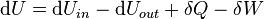$\mathrm{d}U= \mathrm{d}U_{in}-\mathrm{d}U_{out}+\delta Q-\delta W\,$

where Uin is the average internal energy entering the system and Uout is the average internal energy leaving the systemDuring steady, continuous operation, an energy balance applied to an open system equates shaft work performed by the system to heat added plus net enthalpy added.

The region of space enclosed by open system boundaries is usually called a control volume, and it may or may not correspond to physical walls. If we choose the shape of the control volume such that all flow in or out occurs perpendicular to its surface, then the flow of matter into the system performs work as if it were a piston of fluid pushing mass into the system, and the system performs work on the flow of matter out as if it were driving a piston of fluid. There are then two types of work performed: flow work described above which is performed on the fluid (this is also often called$pV$ work) and shaft work which may be performed on some mechanical device.

These two types of work are expressed in the equation: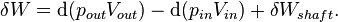$\delta W=\mathrm{d}(p_{out}V_{out})-\mathrm{d}(p_{in}V_{in})+\delta W_{shaft}.\,$

Substitution into the equation above for the control volume cv yields: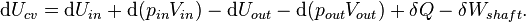$\mathrm{d}U_{cv}=\mathrm{d}U_{in}+\mathrm{d}(p_{in}V_{in}) - \mathrm{d}U_{out}-\mathrm{d}(p_{out}V_{out})+\delta Q-\delta W_{shaft}.\,$

The definition of enthalpy, H, permits us to use this thermodynamic potential to account for both internal energy and$pV$ work in fluids for open systems: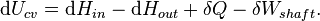$\mathrm{d}U_{cv}=\mathrm{d}H_{in}-\mathrm{d}H_{out}+\delta Q-\delta W_{shaft}.\,$

During steady-state operation of a device (see turbine, pump, and engine), the expression above may be set equal to zero. This yields a useful expression for the power generation or requirement for these devices in the absence of chemical reactions: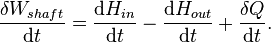$\frac{\delta W_{shaft}}{\mathrm{d}t}=\frac{\mathrm{d}H_{in}}{\mathrm{d}t}- \frac{\mathrm{d}H_{out}}{\mathrm{d}t}+\frac{\delta Q}{\mathrm{d}t}. \,$

This expression is described by the diagram above.

## Standard enthalpy changes

Standard enthalpy changes describe the change in enthalpy observed in the constituents of a thermodynamic system when going between different states under standard conditions. The standard enthalpy change of vaporization, for example gives the enthalpy change when going from liquid to gas. These enthalpies are reversible; the enthalpy change of going from gas to liquid is the negative of the enthalpy change of vaporization. A common standard enthalpy change is the standard enthalpy change of formation, which has been determined for a large number of substances. The enthalpy change of any reaction under any conditions can be computed, given the standard enthalpy change of formation of all of the reactants and products.

### Definitions

#### Chemical Properties

Standard enthalpy change of reaction

 “ Standard enthalpy change of reaction is defined as the enthalpy change observed in a constituent of a thermodynamic system when, one mole of substance reacts completely under standard conditions. ”

Standard enthalpy change of formation

 “ Standard enthalpy change of formation is defined as the enthalpy change observed in a constituent of a thermodynamic system when, one mole of a compound is formed from its elementary antecedents under standard conditions. ”

Standard enthalpy change of combustion

 “ Standard enthalpy of combustion is defined as the enthalpy change observed in a constituent of a thermodynamic system, when one mole of a substance combusts completely with oxygen under standard conditions. ”

Standard enthalpy change of hydrogenation

 “ Standard enthalpy of hydrogenation is defined as the enthalpy change observed in a constituent of a thermodynamic system, when one mole of an unsaturated compound reacts completely with an excess of hydrogen under standard conditions to form a saturated compound. ”

Standard enthalpy change of atomization

 “ Standard enthalpy of atomization is defined as the enthalpy change required to atomize one mole of compound completely under standard conditions. ”

#### Physical Properties

Standard enthalpy change of solution

 “ Standard enthalpy of solution is defined as the enthalpy change observed in a constituent of a thermodynamic system, when one mole of an solute is dissolved completely in an excess of solvent under standard conditions. ”

Standard enthalpy change of fusion

 “ Standard enthalpy of fusion is defined as the enthalpy change required to completely change the state of one mole of substance between solid and liquid states under standard conditions. ”

Standard enthalpy change of vapourization

 “ Standard enthalpy of vapourization is defined as the enthalpy change required to completely change the state of one mole of substance between liquid and gaseous states under standard conditions. ”

Standard enthalpy change of sublimation

 “ Standard enthalpy of sublimation is defined as the enthalpy change required to completely change the state of one mole of substance between solid and gaseous states under standard conditions. ”

Standard enthalpy change of denaturation

 “ Standard enthalpy of denaturation is defined as the enthalpy change required to denature one mole of compound under standard conditions. ”

Lattice enthalpy

 “ Lattice enthalpy is defined as the enthalpy required required to separate one mole of an ionic compound into separated gaseous ions to an infinite distance apart (meaning no force of attraction) under standard conditions. ”

## Specific enthalpy

The specific enthalpy of a working mass is a property of that mass used in thermodynamics, defined as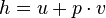$h=u+p \cdot v$ where u is the specific internal energy, p is the pressure, and v is specific volume. In other words,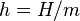$h = H/m$ where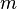$m$ is the mass of the system. The SI unit for specific enthalpy is joules per kilogram.